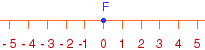Name: Jessica Who is asking: Student Level: Middle Question: F= -3 + 3 WHAT IS F????? Hi Jessica, Think of this as motion along the number. F starts at 0 and moves according to the expression, with + meaning you move to the right and - meaning you move to the left.For example F = 5 - 2 says start at 0, move 5 units to the right and then 2 units to the left. You end up at position 3 and hence F = 5 - 2 = 3 Similarly F = -3 + 4 says start at 0, move 3 units to the left and then 4 units to the right. You end up at position 1 and hence F = -3 + 4 = 1 Now find F = -3 + 3 Cheers, Penny Go to Math Central